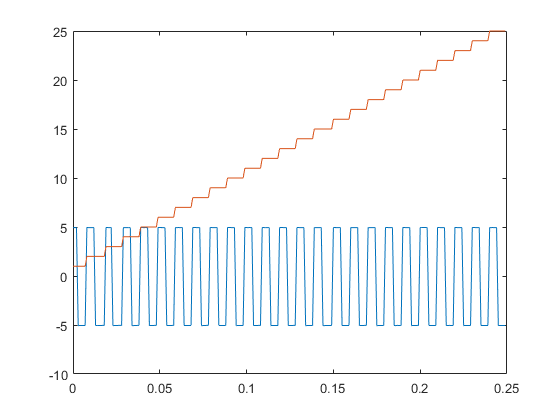# Count Pulses on a Digital Signal Using NI Devices

This example shows how to determine the rate of rotation of an Anaheim Automation motor controller by counting the number of rising edges in the signal. The controller returns hall effect pulses (square waves) that serve as frequency feedback for motor rotation speeds.

### Create a Counter Input Channel

Use `daq` to create a DataAcquisition and `addinput` to add a counter input channel with `EdgeCount` measurement type. For this example, use CompactDAQ chassis NI c9178 and module NI 9402 with ID cDAQ1Mod5.

```dq = daq("ni"); ch = addinput(dq,"cDAQ1Mod5", "ctr0", "EdgeCount"); ch ```
```ch = Index Type Device Channel Measurement Type Range Name _____ ____ ___________ _______ ________________ _____ ________________ 1 "ci" "cDAQ1Mod5" "ctr0" "EdgeCount" "n/a" "cDAQ1Mod5_ctr0" ```

### Determine the Terminal of the Counter Input Channel

To connect the input signal to the correct terminal, examine the `Terminal` property of the channel. The terminal is determined by the hardware.

```ch.Terminal ```
```ans = 'PFI0' ```

To determine if the counter is operational, input a single scan, pause while the motor rotates, then read the counter again.

```read(dq) ```
```ans = timetable Time cDAQ1Mod5_ctr0 _____ ______________ 0 sec 3 ```
```pause(0.1); read(dq) ```
```ans = timetable Time cDAQ1Mod5_ctr0 _____ ______________ 0 sec 14 ```
```pause(0.1); read(dq) ```
```ans = timetable Time cDAQ1Mod5_ctr0 _____ ______________ 0 sec 27 ```

### Measure Revolutions per Second

Count the number of pulses by resetting the counter to zero, pause for one second, and read the counter. The hall effects are oriented every 120 degrees and generate three square wave pulses for every rotation.

```resetcounters(dq); pause(1); read(dq, "OutputFormat", "Matrix")/3 ```
```ans = 33.6667 ```

### Use Hardware Clock for Higher Accuracy

The hardware clock is highly accurate. Use the hardware clock to acquire multiple counter measurements. NI counter devices require an external clock. By adding an analog input channel for a module on the same chassis, the DataAcquisition shares an internal clock with both modules.

```dq = daq("ni"); addinput(dq,"cDAQ1Mod1", "ai0", "Voltage"); addinput(dq,"cDAQ1Mod5", "ctr0", "EdgeCount"); data = read(dq, seconds(0.25)); plot(data.Time, data.Variables); ```